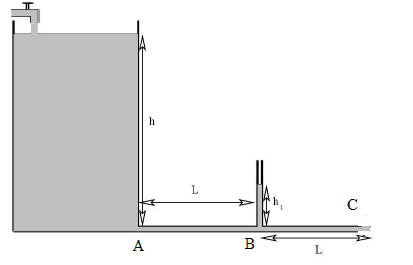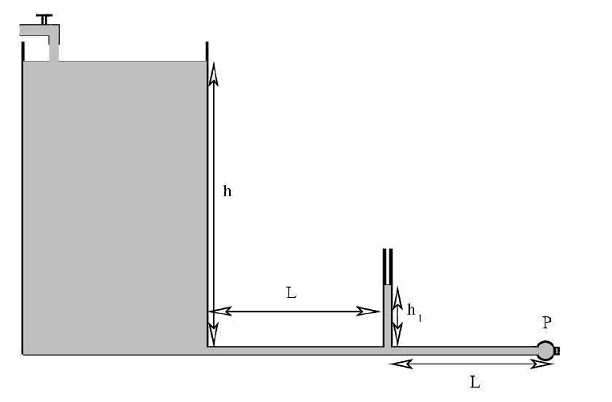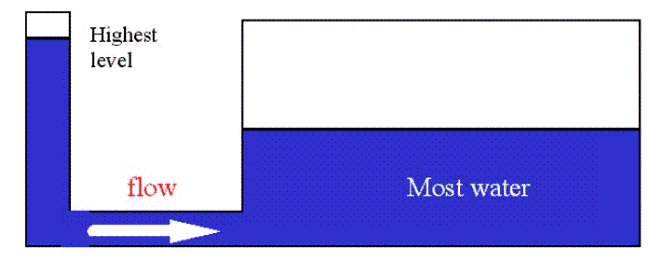# Problem about Hagen-Poiseuille law and pump

• Soren4

## Homework Statement

A tank contains an incompressible liquid of density ##\rho = 1. 4\cdot10^{3}kg / m^3 ## and viscosity ##\eta=1. 4\cdot10^{-3} Pa\cdot s##. The tank is open at the summit and the level of the liquid is kept constant through a sink. At the base of the tank there is a circular pipe, negligible compared to that of the tank, of radius ##r = 0.5 cm## and length ##2L##, ##L = 98 cm##. At the midpoint of a pipe there is a free air vertical manometer that shows a vertical height of the liquid of ##h_1 = 8 cm##.
Determine:
a) the average velocity ##v## of the liquid in the tube;
b) the height ##h## of the liquid in the tub.With the same height of the liquid in the tank, determine
c) what the speed ##v_0## of the liquid in the tube would be if ##\eta=0 Pa \cdot s## (Consider the verical manometer long enough to prevent the liquid to exit from it).
d) the power that the pump ##\mathscr{P}## in the following picture must deliver in order to balance the viscosity and to have, with the value of ##\eta## indicated initially, an average speed of ##v_0## of the liquid in the tube.## Homework Equations

Hagen Pouiseille law $$Q=\frac{\pi r^4 \Delta p}{8\eta L}$$

## The Attempt at a Solution

a) Since I do not know the pressure difference between A and B (I do not know ##h##), I used H.P. law between B and C.Then I used the fact that the fluid is incompressible, which means that ##Q= \pi r^2 v_{average}= \mathrm{constant}##, to find the average speed, so
$$Q=\frac{\pi r^4 [p_{atm}-(p_{atm}+\rho g h_1)] L}{8 \eta L}\implies v_{average}=...$$
b) Then, using again ##Q= \pi r^2 v_{average}= \mathrm{constant}##, I used H.P. law between A and B to find ##h##
$$Q=\frac{\pi r^4 (\rho g h-\rho g h_1)}{8 \eta L}\implies h=...$$
Is the reasoning correct so far?

c)I think that ##v_0=\sqrt{2gh}## but in that case I would have ##h_1=0 m## which is strange, looking at the sentence "Consider the verical manometer long enough to prevent the liquid to exit from it"

d) Here is the most confusing one. I considered the idraulic resistance from H.P. law ##R=\frac{8 \eta L}{\pi r^4}## and I tried with ##\Delta p= Q R## and ##\mathscr{P}=Q \Delta p## ##\implies## ##\mathscr{P}=Q^2 R##

I tried to give an answer to all the points but I do not have the results and I am not convinced of what I have tried. Is there any mistake in what I have written?

Last edited:

## Homework Statement

A tank contains an incompressible liquid of density ##\rho = 1. 4\cdot10^{3}kg / m^3 ## and viscosity ##\eta=1. 4\cdot10^{-3} Pa\cdot s##. The tank is open at the summit and the level of the liquid is kept constant through a sink. At the base of the tank there is a circular pipe, negligible compared to that of the tank, of radius ##r = 0.5 cm## and length ##2L##, ##L = 98 cm##. At the midpoint of a pipe there is a free air vertical manometer that shows a vertical height of the liquid of ##h_1 = 8 cm##.
Determine:
a) the average velocity ##v## of the liquid in the tube;
b) the height ##h## of the liquid in the tub.
View attachment 102021
With the same height of the liquid in the tank, determine
c) what the speed ##v_0## of the liquid in the tube would be if ##\eta=0 Pa \cdot s## (Consider the verical manometer long enough to prevent the liquid to exit from it).
d) the power that the pump ##\mathscr{P}## in the following picture must deliver in order to balance the viscosity and to have, with the value of ##\eta## indicated initially, an average speed of ##v_0## of the liquid in the tube.
View attachment 102023

## Homework Equations

Hagen Pouiseille law $$Q=\frac{\pi r^4 \Delta p}{8\eta L}$$

## The Attempt at a Solution

a) Since I do not know the pressure difference between A and B (I do not know ##h##), I used H.P. law between B and C.Then I used the fact that the fluid is incompressible, which means that ##Q= \pi r^2 v_{average}= \mathrm{constant}##, to find the average speed, so
$$Q=\frac{\pi r^4 [p_{atm}-(p_{atm}+\rho g h_1)] L}{8 \eta L}\implies v_{average}=...$$
b) Then, using again ##Q= \pi r^2 v_{average}= \mathrm{constant}##, I used H.P. law between A and B to find ##h##
$$Q=\frac{\pi r^4 (\rho g h-\rho g h_1)}{8 \eta L}\implies h=...$$
Is the reasoning correct so far?
Your reasoning for part (a) was correct, except for a sign issue. For part (b), the reasoning is not correct. The pressure drop between points A and C is twice the pressure drop between points B and C. So you know the pressure at point A. You now need to apply the Bernoulli equation to the flow in the tank between the surface and point A, taking into account both the difference in elevation and the kinetic energy of the fluid at A (remembering that, in laminar flow, the kinetic energy expression does not have a 2 in the denominator).
c)I think that ##v_0=\sqrt{2gh}## but in that case I would have ##h_1=0 m## which is strange, looking at the sentence "Consider the verical manometer long enough to prevent the liquid to exit from it"
Your reasoning is correct. h1 = 0 in this case.
d) Here is the most confusing one. I considered the idraulic resistance from H.P. law ##R=\frac{8 \eta L}{\pi r^4}## and I tried with ##\Delta p= Q R## and ##\mathscr{P}=Q \Delta p## ##\implies## ##\mathscr{P}=Q^2 R##

I tried to give an answer to all the points but I do not have the results and I am not convinced of what I have tried. Is there any mistake in what I have written?

What does the pressure at point A have to be in order for the velocity in the tube to be v0? Given this result, what does the pressure at point C have to be in order for viscous flow to exist in the region between points A and C at velocity v0?

•Soren4
Thanks a lot for the reply!

I do not understand what you have said about point b. Can I use Bernoulli equation in this case? This is a fluid with nonzero viscosity, so Bernoulli equation's assumptions are not valid, as far as I know.

Anyway if I use Bernoulli equation between a point ##S## at the surface and point ##A## I get

$$p_{atm}+\rho g h= p_a +\frac{1}{2} \rho v^2$$
From here I can get ##v##. (I also don't get why the kinetic energy should not be divided by ##2##).

Finally, If I got that right, the problem with the method for point (b) that I proposed is that ##p_a \neq \rho g h## since the fluid is moving.

Thanks a lot for the reply!

I do not understand what you have said about point b. Can I use Bernoulli equation in this case? This is a fluid with nonzero viscosity, so Bernoulli equation's assumptions are not valid, as far as I know.

Anyway if I use Bernoulli equation between a point ##S## at the surface and point ##A## I get

$$p_{atm}+\rho g h= p_a +\frac{1}{2} \rho v^2$$
From here I can get ##v##. (I also don't get why the kinetic energy should not be divided by ##2##).

Finally, If I got that right, the problem with the method for point (b) that I proposed is that ##p_a \neq \rho g h## since the fluid is moving.
In laminar flow, the velocity profile is not flat. It is parabolic, with twice the average velocity ##\bar{v}## at the center of the tube: $$v=2\bar{v}\left(1-\left(\frac{r}{R}\right)^2\right)$$ So the kinetic energy per unit mass of the flowing fluid in a tube is not ##\bar{v}^2/2##; it works out to be ##\bar{v}^2##. So the application of the Bernoulli equation should be:
$$p_{atm}+\rho g h= p_a + \rho \bar{v}^2$$
The pressure at A should be:
$$p_a=p_{atm}+2(P_B-P_C)$$

Chet

•Soren4
Thanks again for the very clear answer! I think I got the solution of the exercise, could you just specify why the application of Bernoulli equation is valid here? I searched on web and on different books but the condition of ##\eta=0## seems necessary to use Bernoulli equation..

Thanks again for the very clear answer! I think I got the solution of the exercise, could you just specify why the application of Bernoulli equation is valid here? I searched on web and on different books but the condition of ##\eta=0## seems necessary to use Bernoulli equation..
We are only applying the Bernoulli equation up to the exit hole from the tank, and we are neglecting the viscous stresses in the converging flow leading up to the exit hole.

•Soren4
Ok, thanks so much for the answer! I thought about it for a while, If I may ask, it is not clear why the pressure in ##A## cannot be ##\rho g h##. The motion in the tank is neglected since there is a constant re filling of the fluid, so isn't the problem actually about hydrostatics in this case? If so I would use Stevin law, which leads to ##p_a=\rho g h##..

Would you be so kind as to suggest why this reasoning would be wrong? That's a point that really confuses me, since in other similar situations I saw that the pressure at the bottom of a tank with an hole is considered to be ##\rho g h##, as in a hydrostatics problem.

Ok, thanks so much for the answer! I thought about it for a while, If I may ask, it is not clear why the pressure in ##A## cannot be ##\rho g h##. The motion in the tank is neglected since there is a constant re filling of the fluid, so isn't the problem actually about hydrostatics in this case? If so I would use Stevin law, which leads to ##p_a=\rho g h##..

Would you be so kind as to suggest why this reasoning would be wrong? That's a point that really confuses me, since in other similar situations I saw that the pressure at the bottom of a tank with an hole is considered to be ##\rho g h##, as in a hydrostatics problem.
I can help you figure this out yourself. Given that all the flow streamlines have to pass through the exit hole, what do you think the fluid streamline pattern looks like in the region closely approaching the exit hole. (Think of a crowded room in which everyone is trying to get out the door at the same time)

Last edited:
•Soren4
Thanks for the suggestions! Also looking at the question now I see why the pressure in ##A## is not ##\rho g h## because of the velocity of the fluid there.. The streamlines converge in the hole and the fluid gains speed, which means that there is less pressure in the hole.

But I also find a similar question in which there is an example where the pressure in proximity of a hole in a tank is considered to be ##\rho g h##. In that case the hole is not exposed to the atmosphere, but still the pressure there should be different since the fluid is moving. In the question the exercise is solved exactly as I would have solved it, but with the considerations here I'm facing many doubts about it.. Is in that case correct to think at the pressure at the end of the hole as ##\rho g h##?

Thanks for the suggestions! Also looking at the question now I see why the pressure in ##A## is not ##\rho g h## because of the velocity of the fluid there.. The streamlines converge in the hole and the fluid gains speed, which means that there is less pressure in the hole.

But I also find a similar question in which there is an example where the pressure in proximity of a hole in a tank is considered to be ##\rho g h##. In that case the hole is not exposed to the atmosphere, but still the pressure there should be different since the fluid is moving. In the question the exercise is solved exactly as I would have solved it, but with the considerations here I'm facing many doubts about it.. Is in that case correct to think at the pressure at the end of the hole as ##\rho g h##?
The problem is not analyzed correctly in the stackexchange reference (even though it is given a green check by the person asking the question). The people solving this problem tremendously underestimated its complexity. There is no steady state solution to the problem, as implied in the analyses in stackexchange. This is a transient problem, and must be solved using a transient version of the Bernoulli equation. The solution will be an oscillatory function of time, as the heights in the tanks osciallate back and forth forever. Saying that there is a steady state solution to this problem is analogous to saying that there is steady state solution to a spring mass problem in which the system is initially out of equilibrium.

Chet

•Soren4
I have worked out the solution to the stachexchange "a similar question" problem if you would like to see it. But, it is nothing like what you expected.

•Soren4
Thanks so much for your suggestions! If it isn't too much trouble it would be great to see how it is such exercise done correctly, because maybe it would help me and others to clear doubts about it!

Thanks so much for your suggestions! If it isn't too much trouble it would be great to see how it is such exercise done correctly, because maybe it would help me and others to clear doubts about it!This is mainly an "oscillating manometer" problem. The fluid in tank A oscillates up and down, out of phase with the fluid in tank B, which also oscillates up and down.. Fluid flows periodically back and forth between tanks A and B through the connecting channel. This is very much like a spring and mass problem.
Analysis:
Let ##h_A(t)## represent the depth of fluid in tank A at time t
Let ##h_B(t)## represent the depth of fluid in tank B at time t
Let ##A_A## represent the cross sectional area of tank A
Let ##A_B## represent the cross sectional area of tank B
Let ##\rho## represent the density of the fluid

In this analysis, we are going to neglect the kinetic energy of the fluid in the connecting channel (compared to that in the tanks).

Using the base of the tanks as the datum for potential energy, the total potential energy of the fluid in both tanks at any time t is:
$$PE=\rho g A_A\frac{h_A^2}{2}+ \rho g A_B\frac{h_B^2}{2}$$
The total kinetic energy of the fluid in both tanks at any time t is:
$$KE=\frac{\rho A_Ah_A}{2}\left(\frac{dh_A}{dt}\right)^2+\frac{\rho A_Bh_B}{2}\left(\frac{dh_B}{dt}\right)^2$$So the total potential energy plus kinetic energy of the fluid in both tanks at any time t is given by:
$$PE+KE=\rho g A_A\frac{h_A^2}{2}+ \rho g A_B\frac{h_B^2}{2}+\frac{\rho A_Ah_A}{2}\left(\frac{dh_A}{dt}\right)^2+\frac{\rho A_Bh_B}{2}\left(\frac{dh_B}{dt}\right)^2\tag{1}$$
Conservation of mass requires that the total mass of liquid does not change with time. this requires that:
$$h_AA_A+h_BA_B=\bar{h}(A_A+A_B)\tag{2}$$
where ##\bar{h}## is the equilibrium height of the liquid in the two tanks.

This is where I'll stop for now and give you a chance to respond.

Chet

•Soren4
@Soren4 Would you like me to complete this solution, or have you had your fill of this problem?

Here is a reference that does 3 versions of the manometer oscillation problem (depending on the area ratio) and gets the same final results as me, except that the analysis is not as straightforward as my analysis:
[PDF]Manometer Oscillations - Christopher E. Brennen
The third problem that is solved by Brennen is fundamentally identical to the problem in stackexchange.

•Soren4